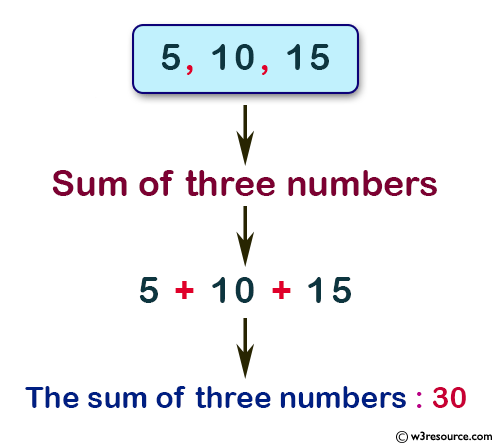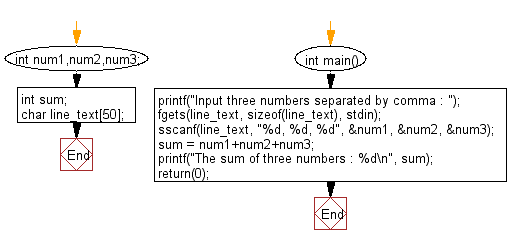﻿ C : Input three numbers in one line and calculate their sum# C Exercises: Calculate the sum of three numbers getting input in one line

## C Input Output statement: Exercise-8 with Solution

Write a program in C to calculate the sum of three numbers with getting input in one line separated by a comma.

Pictorial Presentation:Sample Solution:

C Code:

``````#include <stdio.h>
int num1,num2,num3;         /* declaration of three variables */
int sum;
char line_text;      /* line of input from keyboard */

int main()
{
printf("Input three numbers separated by comma : ");
fgets(line_text, sizeof(line_text), stdin);
sscanf(line_text, "%d, %d, %d", &num1, &num2, &num3);
sum = num1+num2+num3;
printf("The sum of three numbers : %d\n", sum);
return(0);
}
```
```

Sample Output:

```Input three numbers seperated by comma : 5,10,15
The sum of three numbers : 30
```

Flowchart:C Programming Code Editor:

Improve this sample solution and post your code through Disqus.

What is the difficulty level of this exercise?

Test your Programming skills with w3resource's quiz.

﻿